# Square (second power, quadratic) - math problems

#### Number of problems found: 783

• AlexandraAlexandra made a rectangular quilt the measured 3 1/4. 2 3/4 feet in width. What is the area of the quilt in square feet? Write an equation to solve.
• Frame image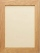Peter wants to put a picture in a frame. The picture is 7 3/5 cm wide. To fit in the picture frame cannot be more than 7 3/10 cm wide. How much should the image be trimmed?
• ParallogramParallogram base is 24 cm and high 10 cm. How many such tile are required to cover a floor of area 1080m2?
• Comparing exponents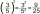A) 8 (6²)(8²) b) 16 (6²)(8²) c) (6¹+²) (8²)
• A trapezoid 3A trapezoid ABCD has the bases length of a = 120 mm, c = 86 mm and the area A = 2,575 mm2. Find the height of the trapezoid.
• Perimeter to area 2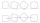Find the area of a square whose perimeter is 100 cm with solve.
• If x=4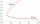If x=4 and y=-3, what is the value of the expression x² + 2xy - 4x + 3y + y²?
• The area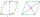The area of a rhombus is 143 m2. If the longer diagonal is 26 m, find the shorter diagonal in inches named d1.
• Backyard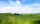Corey's backyard is rectangular with a length of 23 miles. If the area of the backyard is 12 square miles, what is its width?
• Cups of paintI have a board that is 2 meters wide and 3 meters tall. If one cup of paint cover 1,000 square centimeters. How many cups of paints does I need?
• A rectangular 3A rectangular plot of land has an area of 3/2 square kilometers and a length of ¾ kilometer.  What is the width of the plot of land?
• The rugJosie has a rug with the area of 18 square feet. She will put the rug on the floor that is covered in 1/3 square foot tiles. How many tiles will the rug cover?
• Little 2Little fields farm lies on a rectangular plot of land that measures 2 2/4 miles in length and 2 2/3 miles in width. What is it's area in square miles?
• Find the 28Find the area of a square with side length 4/5 yard.
• MohammedMohammed grew a garden last year. His plot of land was 6 3/4 feet by 10 feet. He wants to have twice as much area and his garden this year how much area will the new garden take up?
• Louis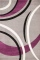Louis wants to carpet the rectangular floor of his basement. The basement has an area of 5,120 square feet. The width of the basement is 4/5 its length. What is the length of Louis's basement?
• AmeekaAmeeka is in charge of designing a school pennant for spirit week. She wants the base to be 3 1/2 feet and the height to be 6 1/2 feet. She has 20 square feet of paper available. Does she have enough paper?
• Fraction unknowns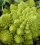Divide of fractions with unknowns: Fraction 1: The quantity x squared plus 6 times x plus 9 over the quantity x minus 1. Fraction 2: the quantity x squared minus 9 over the quantity x squared minus 2 times x plus 1.  Find Fraction 1 over Fraction 2.
• Square area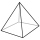Each side of a square is 5 2/3 m. Find the area of the square.
• Base side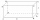In a quadrilateral prism, are known surface area S = 12400 mm2 base side m = 40mm and prism height = 120mm, what is the length of base side n =?

Do you have an exciting math question or word problem that you can't solve? Ask a question or post a math problem, and we can try to solve it.

We will send a solution to your e-mail address. Solved examples are also published here. Please enter the e-mail correctly and check whether you don't have a full mailbox.

Please do not submit problems from current active competitions such as Mathematical Olympiad, correspondence seminars etc...